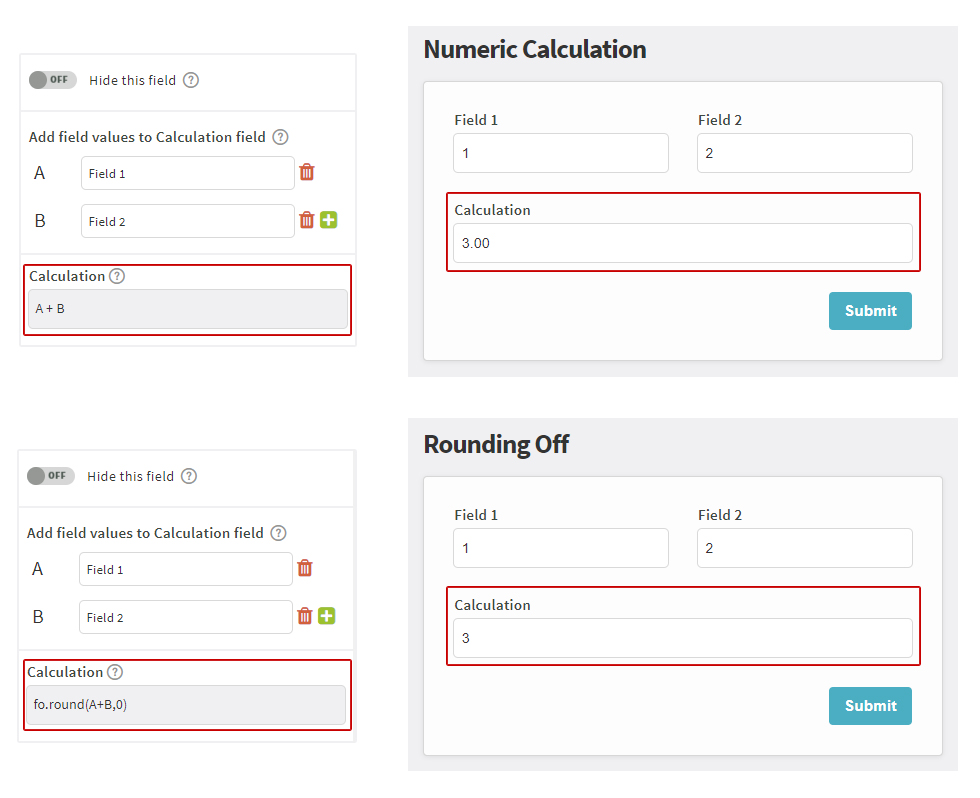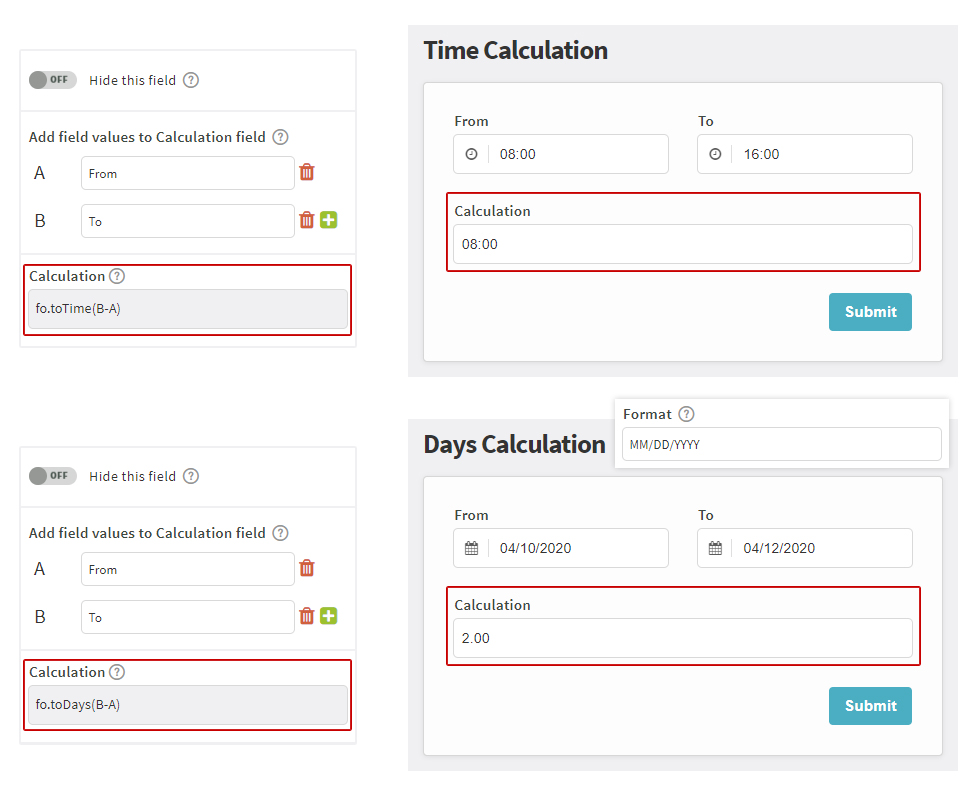# Perform Calculations

• Support
• Account
• Integration
• Manage Formlet
• Others

Formlets makes it easy to compute field values based on user input.

Numeric Calculations
1. Add Number fields, and a Calculation field
2. Disable Hide this field
3. Add field values to the Calculation fieldBy default, calculation is rounded to two decimal places. To round your total to the nearest whole number, set calculation field to fo.round(A+B,0). You may replace zero to the number of decimal places you want to round off.

Date/Time Calculations
1. Add Date or Time fields, and a Calculation field
2. Disable Hide this field
3. Set Calculation field to fo.toTime(B-A) for time, and fo.toDays(B-A) for calculating days